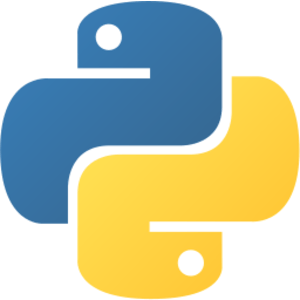# Decorator 概念簡介## 前言

def a_function_wrapper(inner_function):
def wrapper(*args, **kwargs):
print("The function starts!")
returned_values = \
inner_function(*args, **kwargs)
print("The function ends!")
return returned_values
return wrapper

@a_function_wrapper
def original_function():
print("I run normally~")

if __name__ == "__main__":
original_function()


## 為什麼我們需要 decorator 呢？

def i_am_a_dog():
print("I am a dog.")

return x + y

if __name__ == "__main__":
# 「好麻煩！」那幾行都是為了這情境加上去的，\
#     本來只要中間那行
print("Function starts!")  # 好麻煩！
i_am_a_dog()
print("Function ends!")    # 好麻煩！

print("Function starts!")  # 好麻煩！
print("Function ends!")    # 好麻煩！


print("I am a dog.")


print("Function starts!")  # 好麻煩！
# vvvvvv 注意下面都不會動！ vvvvvv
print("I am a dog.")
# ^^^^^^ 注意上面都不會動！ ^^^^^^
print("Function ends!")    # 好麻煩！


print("Function starts!")  # 好麻煩！
# vvvvvv 注意下面都不會動！ vvvvvv
i_am_a_dog()
# ^^^^^^ 注意上面都不會動！ ^^^^^^
print("Function ends!")    # 好麻煩！


function_to_be_changed = i_am_a_dog
print("Function starts!")  # 好麻煩！
# vvvvvv 注意下面都不會動！ vvvvvv
function_to_be_changed()
# ^^^^^^ 注意上面都不會動！ ^^^^^^
print("Function ends!")    # 好麻煩！


### functions as variables

def func(a, b, c):
# do something
pass


func = ...


func = lambda a, b, c: pass


### 傳遞參數的本質

def adder(x, y):
return x + y

c = adder(3, 4)  # 注意我！我等等會被解體 Q_Q


x, y = 3, 4  # 我就是函數括號裡的東西啦！
# 接著函數到 return 以前的東東，但 adder 沒有
c = x + y  # 原本函數的位子就換成 return 後面接的東西囉～


### ___

function_to_be_changed = i_am_a_dog  # --> 這邊變成 \
# 新函數的 input

print("Function starts!")  # 好麻煩！
# vvvvvv 注意下面都不會動！ vvvvvv
function_to_be_changed()
# ^^^^^^ 注意上面都不會動！ ^^^^^^
print("Function ends!")    # 好麻煩！

i_am_a_dog = function_to_be_changed  # --> 這邊是 \
# 放回原位的動作


def function_wrapper(function_to_be_changed):
print("Function starts!")  # 好麻煩！
# vvvvvv 注意下面都不會動！ vvvvvv
function_to_be_changed()
# ^^^^^^ 注意上面都不會動！ ^^^^^^
print("Function ends!")    # 好麻煩！

return function_to_be_changed  # --> 很重要！這邊 \
# 是放回原位的動作！

i_am_a_dog = function_wrapper(i_am_a_dog)


function_to_be_changed = adder  # --> 這邊變成 \
# 新函數的 input

print("Function starts!")  # 好麻煩！
# vvvvvv 注意下面都不會動！ vvvvvv
function_to_be_changed()
# ^^^^^^ 注意上面都不會動！ ^^^^^^
print("Function ends!")    # 好麻煩！

adder = function_to_be_changed  # --> 這邊是 \
# 放回原位的動作


### *args 用法

x = 3, 4, 5


def variable_input(x):
for i in x:
print(i)

variable_input([3, 4, 5])  # 用 list
variable_input((3, 4, 5))  # 用 tuple


                         # 自動幫你把所有 inputs \
def variable_input(*x):  # 打包成 tuple x
for i in x:
print(i)

variable_input(3, 4, 5)


（**kwargs 是對 func(x=3, y=4) 這種情況設計的，容我以後有機會再討論，但原理其實相似）

### ___

args = x, y                 # 這些是 \
# function_to_be_changed \
# 的參數們
function_to_be_changed = adder  # --> 這邊變成 \
# 新函數的 input

# ----------- 以下是新函數的內部 ----------- #

print("Function starts!")  # 好麻煩！
# vvvvvv 注意下面都不會動！ vvvvvv
output = function_to_be_changed(*args)
# ^^^^^^ 注意上面都不會動！ ^^^^^^
print("Function ends!")    # 好麻煩！

# ----------- 以上是新函數的內部 ----------- #

wrapper_output = output         # 內部的 output， \
# 不知道塞哪裡欸？
adder = function_to_be_changed  # --> 這邊是 \
# 放回原位的動作


adder = new_function(adder)


args = x, y                 # 這些是 \
# function_to_be_changed \
# 的參數們
function_to_be_changed = adder  # --> 這邊變成 \
# 新函數的 input

# ----------- 以下是新函數的內部 ----------- #

def some_process(*args):
print("Function starts!")  # 好麻煩！
# vvvvvv 注意下面都不會動！ vvvvvv
output = function_to_be_changed(*args)
# ^^^^^^ 注意上面都不會動！ ^^^^^^
print("Function ends!")    # 好麻煩！
return output

# ----------- 以上是新函數的內部 ----------- #

adder_new = some_process  # --> 這邊是 \
# 放回原位的動作

# 所以便會啟動所有包裝過的動作，
# 然後把 output 放在 wanted_output，達到目標


args = x, y
def new_function(function_to_be_changed):

# ----------- 以下是新函數的內部 ----------- #

def some_process(*args):
print("Function starts!")  # 好麻煩！
# vvvvvv 注意下面都不會動！ vvvvvv
output = function_to_be_changed(*args)
# ^^^^^^ 注意上面都不會動！ ^^^^^^
print("Function ends!")    # 好麻煩！
return output

# ----------- 以上是新函數的內部 ----------- #

return some_process  # --> 這邊是 \
# 放回原位的動作

# 所以便會啟動所有包裝過的動作，
# 然後把 output 放在 wanted_output，達到目標


adder = new_function(adder)  # <-- 注意！我之前出現過！
# 要使用時：
# >>> 方法一
# >>> 方法二
wanted_output = adder(x, y)  # 不用多個 args 也可以～


i_am_a_dog = new_function(i_am_a_dog)


@new_function
def i_am_a_dog():
# ...
pass


def 被包裝函數():
pass

def 裝飾器(某函數):
# something
def 替身函數():  # <-- 作為被包裝函數的替身
某函數()
# something
return 替身函數Amah